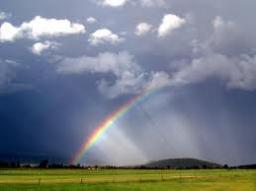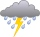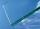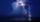# Rainfall

Annual rainfall in our country is an average of 797 mm. How many m3 of water rains on average per hectare?

V =  7970 m3

### Step-by-step explanation:Did you find an error or inaccuracy? Feel free to write us. Thank you!Tips to related online calculators
Do you want to convert area units?
Do you want to convert length units?
Do you know the volume and unit volume, and want to convert volume units?

#### You need to know the following knowledge to solve this word math problem:

We encourage you to watch this tutorial video on this math problem:

## Related math problems and questions:

• Annual rainfallThe average annual rainfall is 686 mm. How many liters will fall on the 1-hectare field?
• RainfallHow many liters of water did fell in a 32m long and 8m wide garden, if 8mm of rain fell?
• RainfallThe annual average rainfall in India was in Cherrapunji in the year 1981 26 461 mm. How many hectoliters of water fell on 1 m2? Would fit this amount of water into a cube of three meters?
• RainfallA rectangular garden of 25m in length and width 20m in width fall 4mm of water. Express by a fraction in basic form what part of the 60-hectolitre tank we would fill with this water.
• RainfallOn Thursday, fell 1 cm rainfall. How many liters of water fell to rectangular garden with dimensions of 22 m x 35 m?
• Tank full2 hl of water were poured into a block-shaped tank with dimensions of 450 mm and 600 mm and a height of 900 mm. How many liters of water need to be added to keep the tank full?
• GardenGarden with an area of 800 square meters rained 3 mm of water. How many 10-liter cans of water is needed to same irrigation?
• The potThe pot is in 1/3 filled with water. Bottom of the pot has an area of ​​329 cm2. How many centimeters rises water level in the pot after add 1.2 liters of water?
• RainGarden shape of a rectangle measuring 15 m and 20 m rained water up to 3 mm. How many liters of water rained in the garden?
• GlassPeter broke the window glass with size 110 cm and 90 cm. 1 square meter of glass costs 11 USD. How much money is need to pay for a new glass?
• Surface and volume od cuboidThe content area of the square base of the cuboid is Sp = 36 cm2, and its height is 80 mm. Determine its surface area and volume.
• The cuboidThe cuboid is full to the brim with water. The external dimensions are 95 cm, 120 cm, and 60 cm. The thickness of all walls and the bottom is 5 cm. How many liters of water fit into the cuboid?
• The aquariumThe aquarium is 120 cm long, 70 cm wide and 96 cm high. How high does the water reach if they filled 168l of water into the aquarium?
• Four prismsQuestion No. 1: The prism has the dimensions a = 2.5 cm, b = 100 mm, c = 12 cm. What is its volume? a) 3000 cm2 b) 300 cm2 c) 3000 cm3 d) 300 cm3 Question No.2: The prism base is a rhombus with a side length of 30 cm and a height of 27 cm. The height of t
• Swimming pool 4The pool shaped cuboid measuring 12.5 m × 640 cm at the bottom is 960hl water. To what height in meters reaches the water level?
• The tankThe tank has 1320 liters of water. The tank has the shape of a prism, its base is an rectangle with sides a = 0,6 m and b = 1,5 m. How high does the water level reach in the tank?
• WaterOn the lawn which has area 914 m2 rained 3 mm of water. How many liters of water rained?